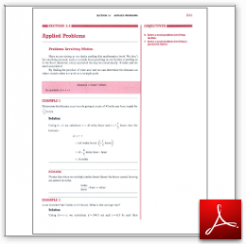Algebra Math Book - Introductory Algebra Thank you for your continual support.

 The power of mathematics rests in the logic of thinking.ID: Sec3-5
Description: Problems Involving Motion-Geometric Figures
Price: 1.99

# Algebra Math book - Introductory Algebra - Chapter 3 - Section 5 - Applied Problems

## Section 3.5 - Objectives

6.  Solve a word problem involving motion.

7.  Solve a word problem involving a geometric figure.

Navigate to
Previous Section or Next Section
or Chapter 3 Details or Other Chapters

This section of my algebra math book, Introductory Algebra, also includes in the download:

• Cover Sheet